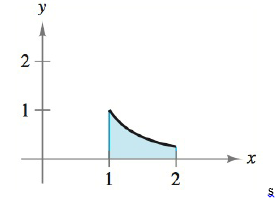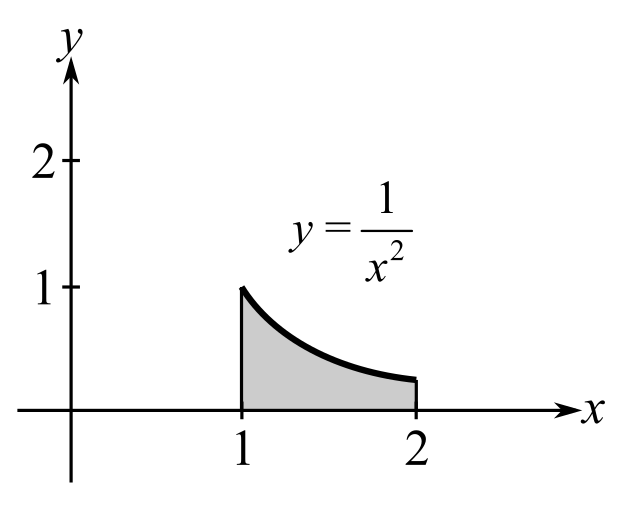Chapter 5.4, Problem 11E### Calculus: An Applied Approach (Min...

10th Edition
Ron Larson
ISBN: 9781305860919

#### Solutions

Chapter
Section### Calculus: An Applied Approach (Min...

10th Edition
Ron Larson
ISBN: 9781305860919
Textbook Problem
1 views

# Finding Area by the Fundamental Theorem In Exercises 9-16, find the area of the region. See Example 2. y = 1 x 2To determine

To calculate: The area of the region y=1x2 in the provided figure.Explanation

Given Information:

The function is y=1x2 and the provided figure is:

Formula used:

The fundamental theorem of calculus states that,

If f is integrable on interval [a,b] then abf(x)dx=F(b)F(a).

The integration formula is xndx=xn+1n+1+C.

Calculation:

Consider the figure.

To find the area of the region, fundamental theorem of calculus can be used.

Integrate the function from x=1 to x=2 as shown below,

12(1x2)dx

This can be written as,

12(1x2)dx=12(x2

### Still sussing out bartleby?

Check out a sample textbook solution.

See a sample solution

#### The Solution to Your Study Problems

Bartleby provides explanations to thousands of textbook problems written by our experts, many with advanced degrees!

Get Started

#### Find more solutions based on key concepts